Next: Non-Degenerate Perturbation Theory Up: Time-Independent Perturbation Theory Previous: Improved Notation

# Two-State System

Consider the simplest possible non-trivial quantum mechanical system. In such a system, there are only two independent eigenstates of the unperturbed Hamiltonian: i.e.,(875)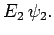(876)

It is assumed that these states, and their associated eigenvalues, are known. We also expect the states to be orthonormal, and to form a complete set.

Let us now try to solve the modified energy eigenvalue problem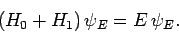(877)

We can, in fact, solve this problem exactly. Since the eigenstates of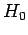form a complete set, we can write [see Eq. (865)](878)

It follows from (877) that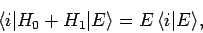(879)

whereor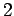. Equations (875), (876), (878), (879), and the orthonormality condition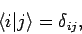(880)

yield two coupled equations which can be written in matrix form: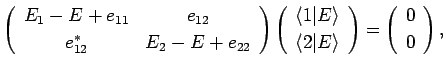(881)

where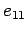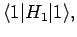(882)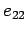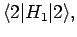(883)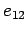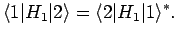(884)

Here, use has been made of the fact thatis an Hermitian operator.

Consider the special (but not uncommon) case of a perturbing Hamiltonian whose diagonal matrix elements are zero, so that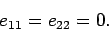(885)

The solution of Eq. (881) (obtained by setting the determinant of the matrix to zero) is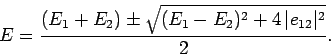(886)

Let us expand in the supposedly small parameter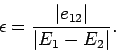(887)

We obtain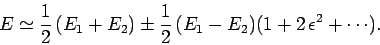(888)

The above expression yields the modification of the energy eigenvalues due to the perturbing Hamiltonian: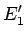(889)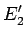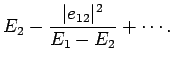(890)

Note thatcauses the upper eigenvalue to rise, and the lower to fall. It is easily demonstrated that the modified eigenstates take the form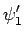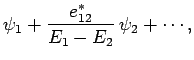(891)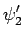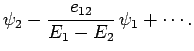(892)

Thus, the modified energy eigenstates consist of one of the unperturbed eigenstates, plus a slight admixture of the other. Now our expansion procedure is only valid when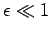. This suggests that the condition for the validity of the perturbation method as a whole is(893)

In other words, when we say thatneeds to be small compared to, what we are really saying is that the above inequality must be satisfied.Next: Non-Degenerate Perturbation Theory Up: Time-Independent Perturbation Theory Previous: Improved Notation
Richard Fitzpatrick 2010-07-20